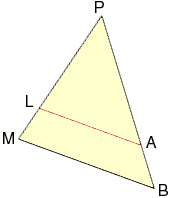My question... Consider 2 triangles: Triangle PMB and Triangle PLA. Triangle PLA is contained within Triangle PMB. Side LA is parallel to Side MB. Point L is located on Side PM. Point A is located on Side PB. If the ratio PL:LM = 5, then what is PB:PA ?? My answer key says 8/5 but I can't explain why. Hi Scott, My diagram of what you describe is below.Triangles PMB and PLA are similar so if PL:LM = 5:1 then PA:AB = 5:1. Hence PB:PA = 6:5. It looks to me as if there is a typo in the problem. I expect that it should say PL:LM = 5:3 Harley Go to Math Central